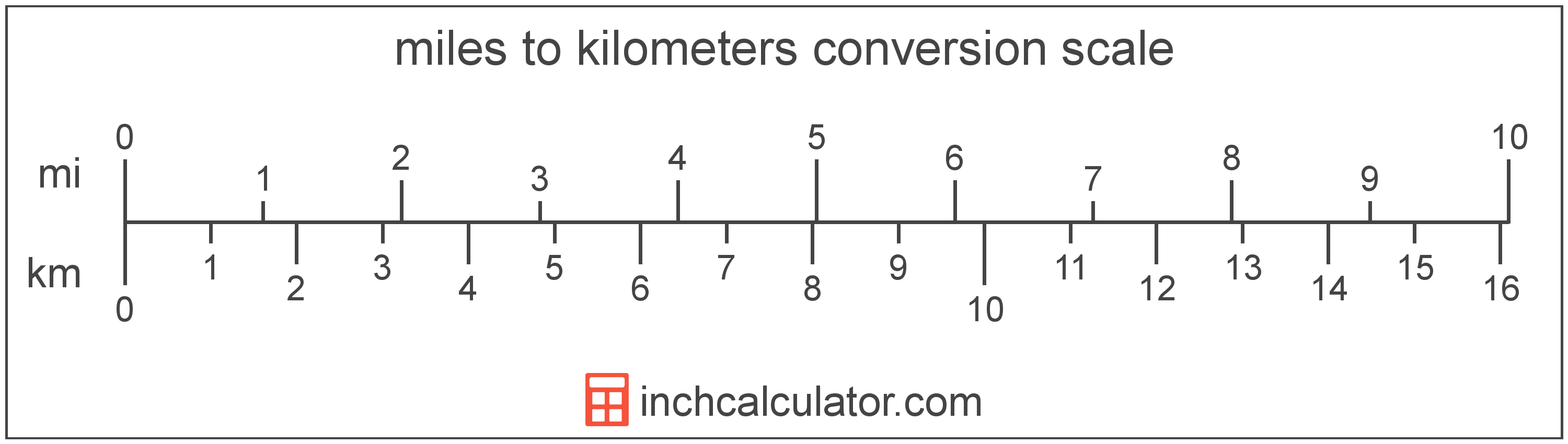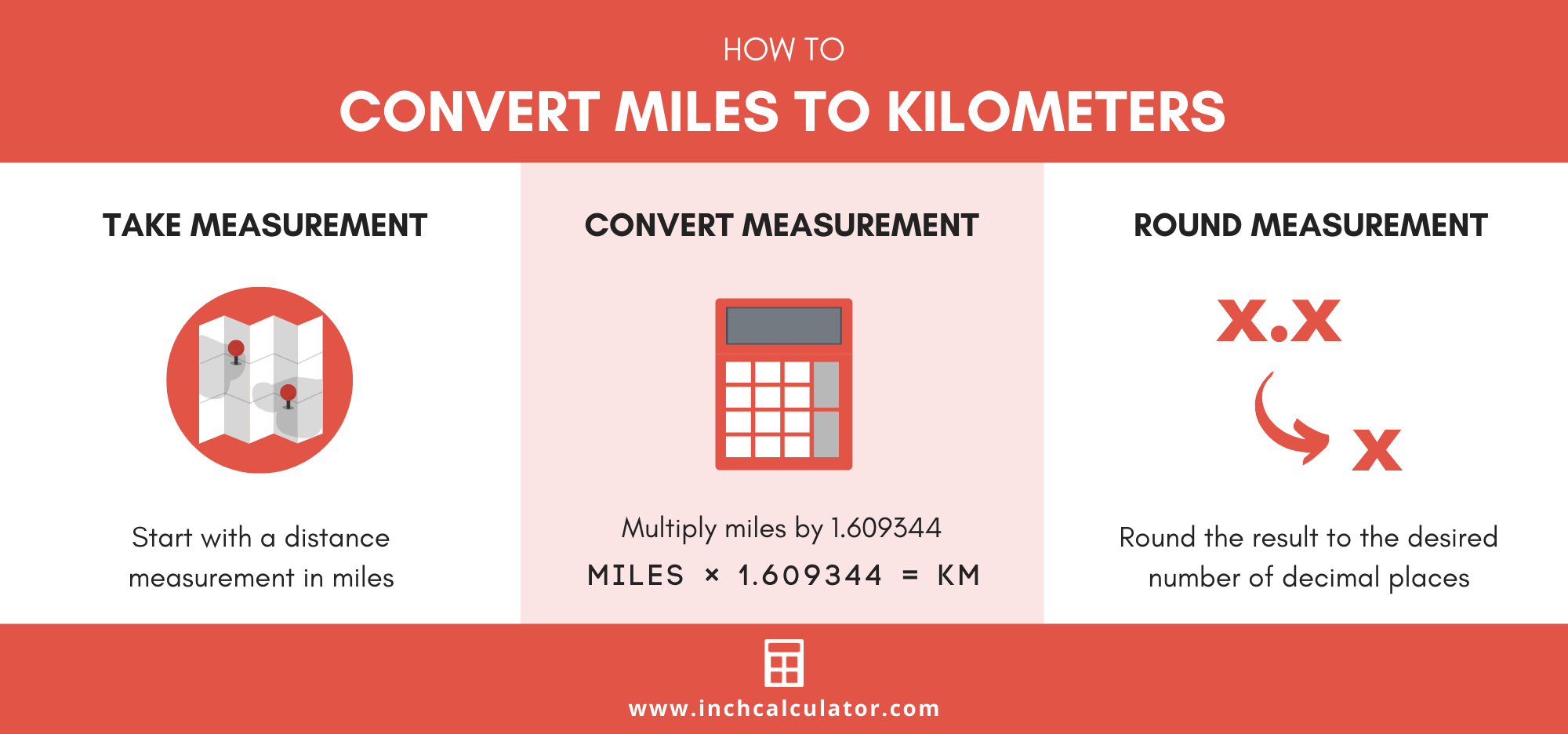# Miles To Km Converter (Miles To Kilometers)

Enter the length in miles below to get the value converted to kilometers.

Results in Kilometers:1 mi = 1.609344 km

Do you want to convert kilometers to miles?

## How to Convert Miles to Kilometers

The fastest way to convert miles to km (kilometers) is to use this simple formula:

kilometers = miles × 1.609344

The distance in kilometers is equal to the distance in miles multiplied by 1.609344. Since one mile is equal to 1.609344 kilometers, that's the conversion ratio used in the formula.

For example, here's how to convert 5 miles to kilometers using the formula above.

kilometers = (5 mi × 1.609344) = 8.04672 km### How Many Kilometers Are in a Mile?

There are 1.609344 kilometers in a mile, which is why we use this value in the formula above.

1 mi = 1.609344 km

## How to Convert Miles to Kilometers Without a Calculator

Multiplying by the conversion ratio of 1.609344 might be quick using a calculator, but what if you want to convert miles to kilometers without one?

While it's not nearly as accurate, there's actually a pretty easy method for converting in your head that will get you close.

multiply miles by 1 (50 x 1 = 1) multiply miles by .6 (50 x .6 = 5 x 6 = 30) add them together (50 + 30 = 80 mi) Follow these three steps to convert miles to kilometers:

• Multiply miles by 1
• Multiply miles by .6
• Add these two values together

For example, here's how to convert 50 miles to kilometers using this method.

• 50 mi × 1 = 50
• 50 mi × .6 = 5 × 6 = 30
• 50 + 30 = 80 km

The result of 80 km is pretty close to the actual value of 80.4672 and could be done pretty quickly. Bottom line, if you need precision, use the calculator above, but this method will save you some time!## What Is a Mile?

The mile is a linear measurement of length equal to exactly 1,609.344 meters or 1.60934 kilometers. One mile is also equal to 5,280 feet or 1,760 yards.

The mile is a US customary and imperial unit of length. Miles can be abbreviated as mi, and are also sometimes abbreviated as m. For example, 1 mile can be written as 1 mi or 1 m.

## What Is a Kilometer?

One kilometer is equal to 1,000 meters, which are defined as the distance light travels in a vacuum in 1/299,792,458 of a second. One kilometer is equal to 0.621371 miles.

The kilometer, or kilometre, is a multiple of the meter, which is the SI base unit for length. In the metric system, "kilo" is the prefix for thousands, or 103. Kilometers can be abbreviated as km; for example, 1 kilometer can be written as 1 km.

## Mile to Kilometer Conversion Table

Table showing various mile measurements converted to kilometers.
Miles Kilometers
1 mi 1.6093 km
2 mi 3.2187 km
3 mi 4.828 km
4 mi 6.4374 km
5 mi 8.0467 km
6 mi 9.6561 km
7 mi 11.27 km
8 mi 12.87 km
9 mi 14.48 km
10 mi 16.09 km
11 mi 17.7 km
12 mi 19.31 km
13 mi 20.92 km
14 mi 22.53 km
15 mi 24.14 km
16 mi 25.75 km
17 mi 27.36 km
18 mi 28.97 km
19 mi 30.58 km
20 mi 32.19 km
21 mi 33.8 km
22 mi 35.41 km
23 mi 37.01 km
24 mi 38.62 km
25 mi 40.23 km
26 mi 41.84 km
27 mi 43.45 km
28 mi 45.06 km
29 mi 46.67 km
30 mi 48.28 km
31 mi 49.89 km
32 mi 51.5 km
33 mi 53.11 km
34 mi 54.72 km
35 mi 56.33 km
36 mi 57.94 km
37 mi 59.55 km
38 mi 61.16 km
39 mi 62.76 km
40 mi 64.37 km

## References

1. Kenneth Butcher, Linda Crown, & Elizabeth J. Gentry, The International System of Units (SI) – Conversion Factors for General Use, National Institute of Standards and Technology, https://nvlpubs.nist.gov/nistpubs/Legacy/SP/nistspecialpublication1038.pdf
2. Ambler Thompson and Barry N. Taylor, Guide for the Use of the International System of Units (SI), National Institute of Standards and Technology, https://physics.nist.gov/cuu/pdf/sp811.pdf